Next: Dirac delta function Up: What is Green's Function Previous: Another Interpretation of G

# PROPERTIES OF THE GF (HEAT EQUATION).

The following properties are common to Green's functions for the heat equation on domain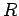.

1. Auxiliary problem.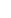Every GF satisfies an auxiliary problem, which includes a Dirac delta generation term in the differential equation, homogeneous boundary conditions of the same type as the original boundary value problem, and a homogeneous initial condition.

2. Causality.In domain,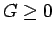for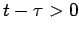, and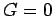for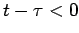. This is called the causality relation, because the GF exhibits zero response until after the heat impulse appears.

3. Reciprocity.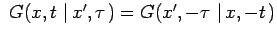. This follows from the heat equation which is second order in space and first order in time.

4. Time dependence. The time dependence of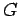is always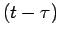, so the functional form of a one-dimensional GF could be written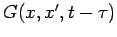.

5. Units. The transient GF takes its units from the (spatial) Dirac delta function, which depends on the dimensionality of the problem. For the heat equation in rectangular coordinates,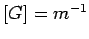for one-dimensional problems,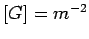for two-dimensional problems, and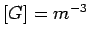for three-dimensional problems.Next: Dirac delta function Up: What is Green's Function Previous: Another Interpretation of G
2004-01-21